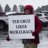#### TonXas

##### New member
VIP
How do I incorporate the average type function into my averages? I tried changing `def HSMA2 = average(dayHigh[-displace], length2);` to `def HSMA2 = averagetype(dayHigh[-displace], length2);` and that didnt work.

Code:
``````input aggregationPeriod = AggregationPeriod.Four_hours;
def len = 2;
input length2 = 2;
input length5 = 5;
input length10 = 10;
input displace = 0;
input averagetype = {default Wilders, EMA, sma, Hull};
def dayHigh = DailyHighLow(aggregationPeriod, len, 0, no).dailyhigh;
def dayLow = DailyHighLow(aggregationPeriod, len, 0, no).DailyLow;
def Dayopen = open(period = aggregationPeriod);
def na = Double.NaN;
def HSMA2 = average(dayHigh[-displace], length2);
def LSMA2 = Average(dayLow[-displace], length2);
def ADR2 = (HSMA2 - LSMA2);
def HSMA5 = Average(dayHigh[-displace], length5);
def LSMA5 = Average(dayLow[-displace], length5);
def ADR5 = (HSMA5 - LSMA5);
def HSMA10 = Average(dayHigh[-displace], length10);
def LSMA10 = Average(dayLow[-displace], length10);
def ADR10 = (HSMA10 - LSMA10);

Last edited by a moderator:##### Active member
VIP
AverageType is a Constant, not a Function...In addition, Average() does not accept AverageType as it is a simple average only...

Edited to add: If you want to implement AverageType you should use MovingAverage()...

Last edited:#### TonXas

##### New member
VIP
AverageType is a Constant, not a Function...How would I rewrite the script in order to be able to switch average types?##### Active member
VIP
How would I rewrite the script in order to be able to switch average types?
I was adding to my previous reply while you were posting this...Thinkscript for Moving Average from different Index/Indicator Questions 2